Walkthroughs

# Get all the keys in Uncharted: Fortune Hunter - Pizarro's Skull Cup 31-40

Gotta catch 'em all

|
| Uncharted: Fortune Hunter

Having trouble getting all the keys in Uncharted: Fortune Hunter? Don't worry, we've got your back. Follow these guides and you'll have them all in no time flat.

Level 31

First move here.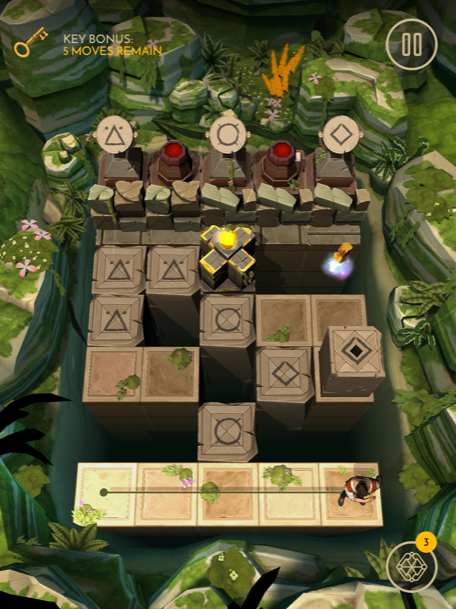Then move here.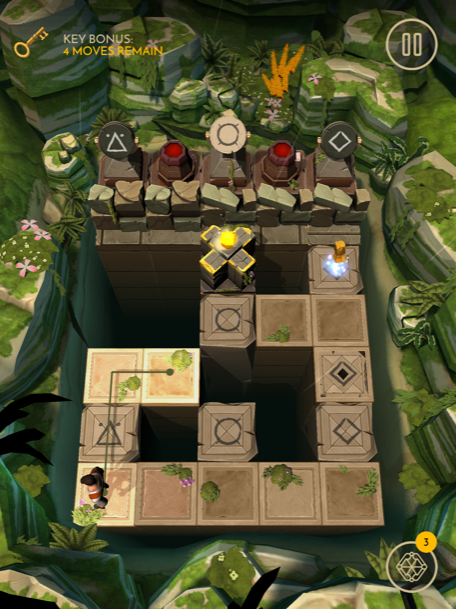Next move here.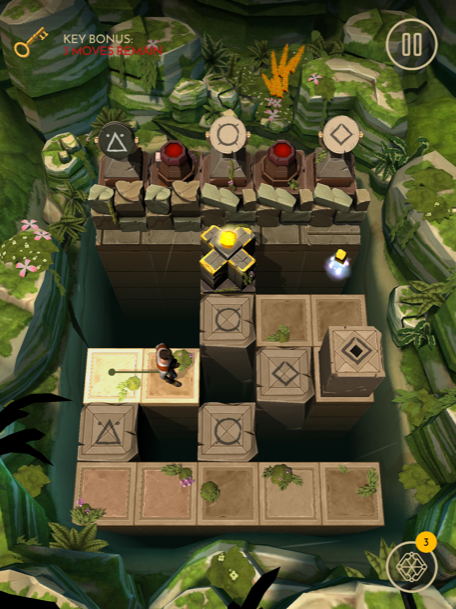Follow this path to the treasure.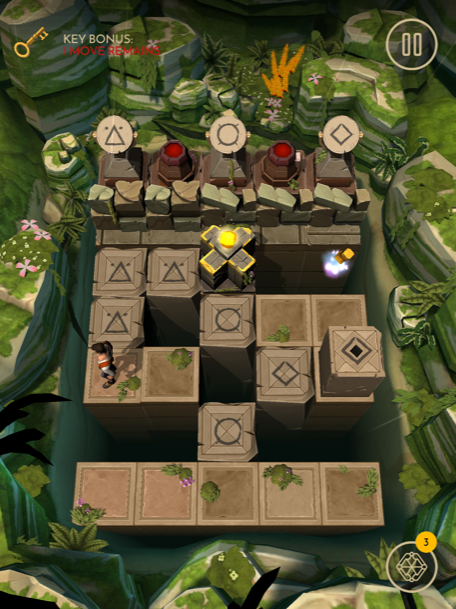Level 32

First move here and push the switch.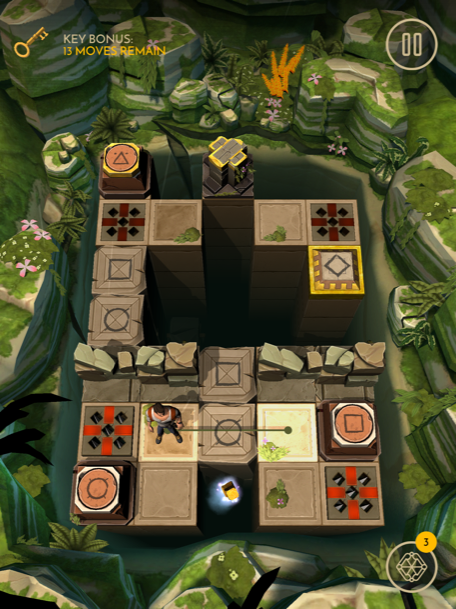Shoot the bomb, then move here.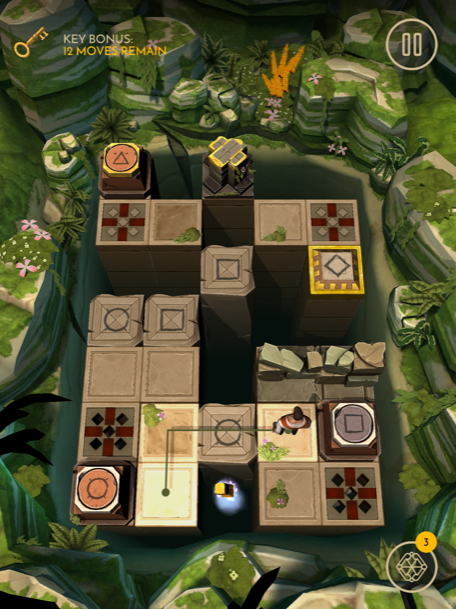Push the switch, then move here.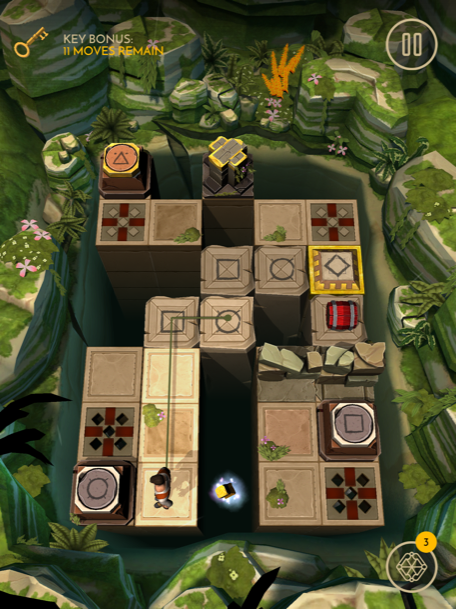Shoot the bomb, then move here.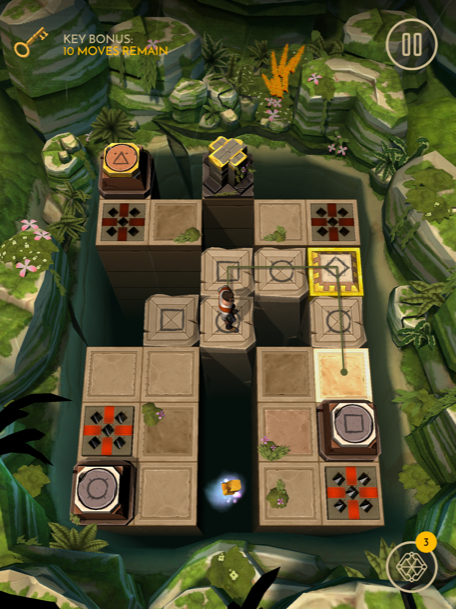Push the switch, then move here.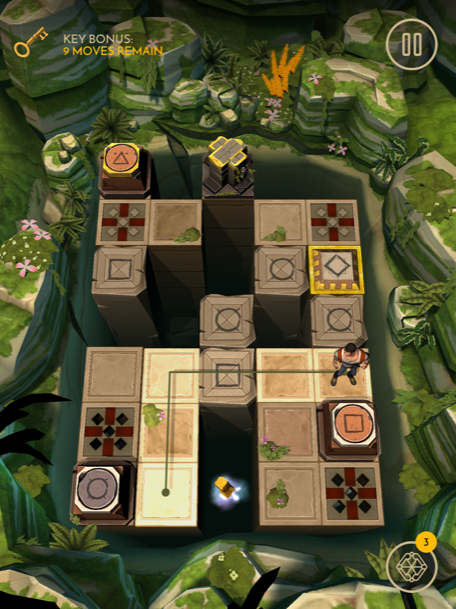Push the switch, then move here.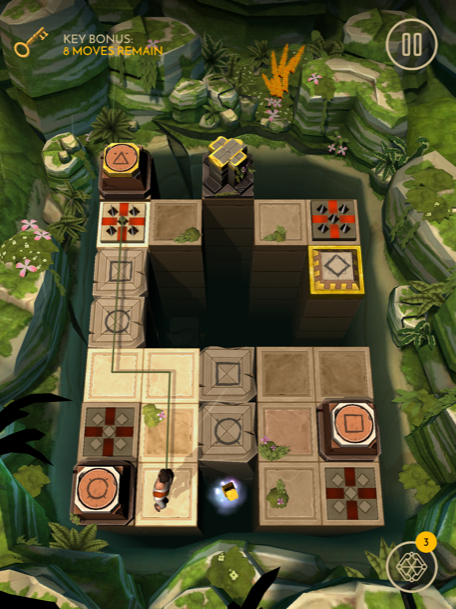Push the switch, then move here.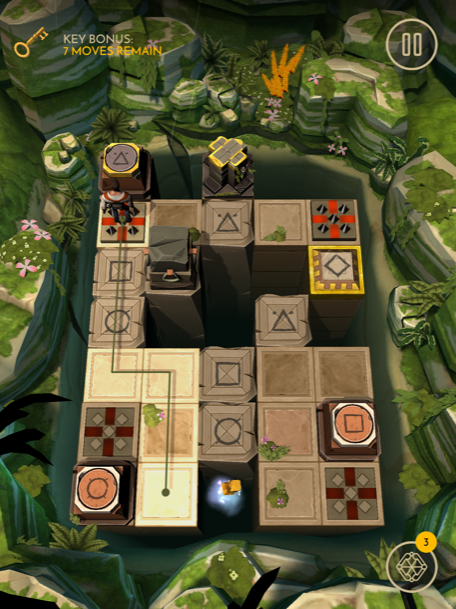Push this switch, then move here.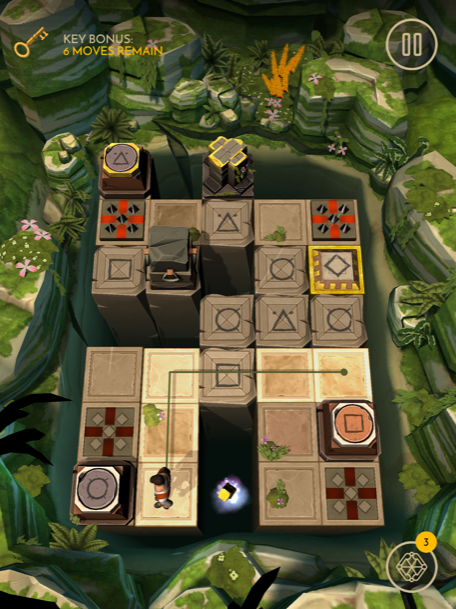Now push this switch. Move here.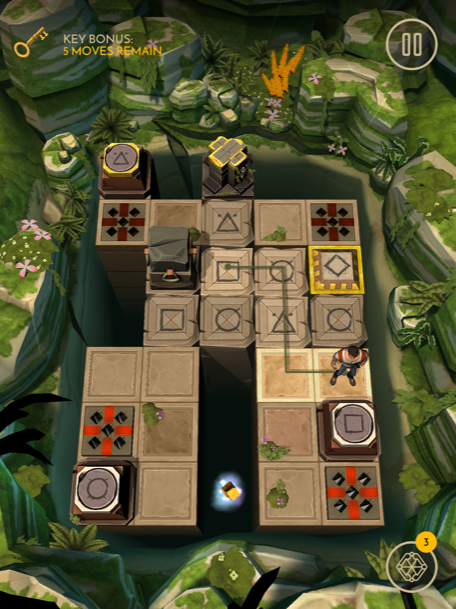Tap the block and drag it here.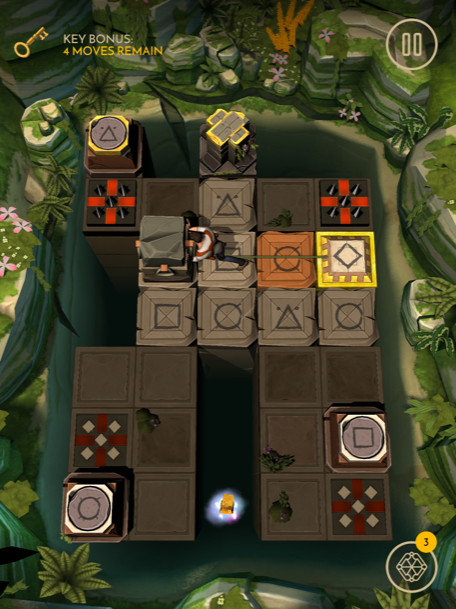Move here, then tap the block.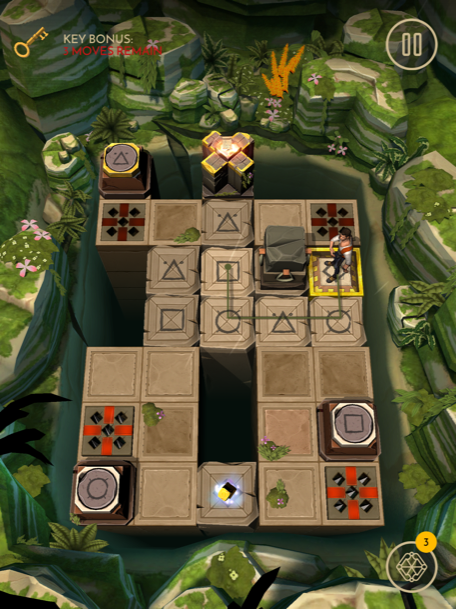Push the block here.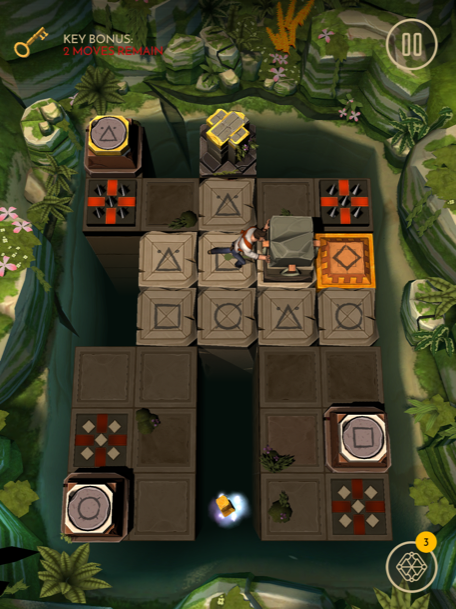Now follow this path and grab the treasure.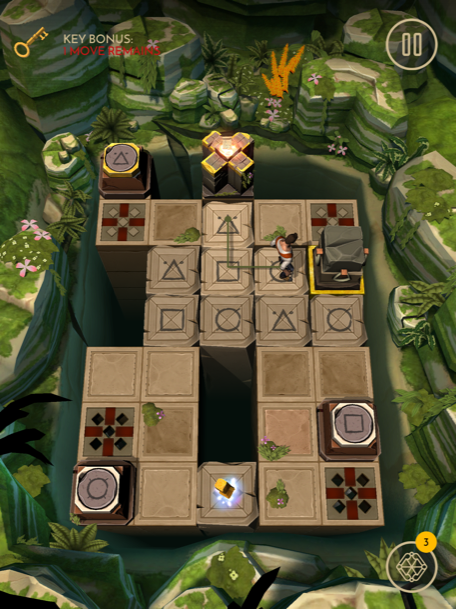Level 33

First pick up the bomb directly above you and place it here.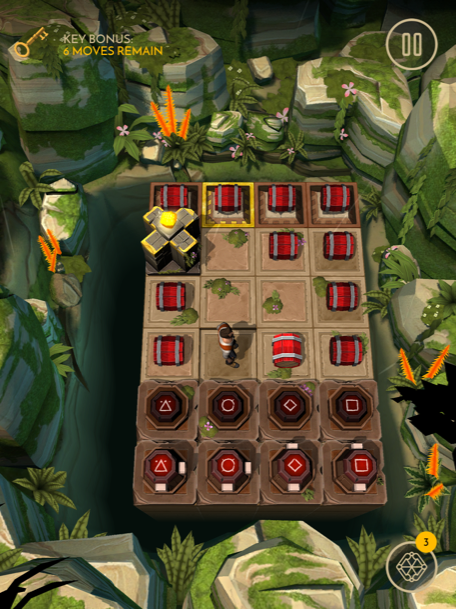Now pick up the bomb behind you and move here.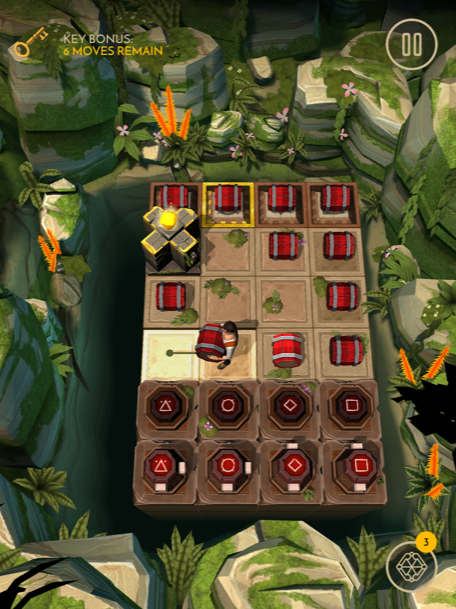Move here, then drop the bomb in front of you.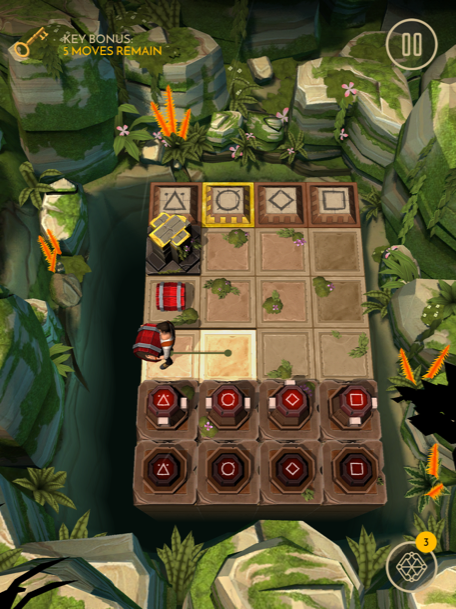Now move here and pick up the bomb.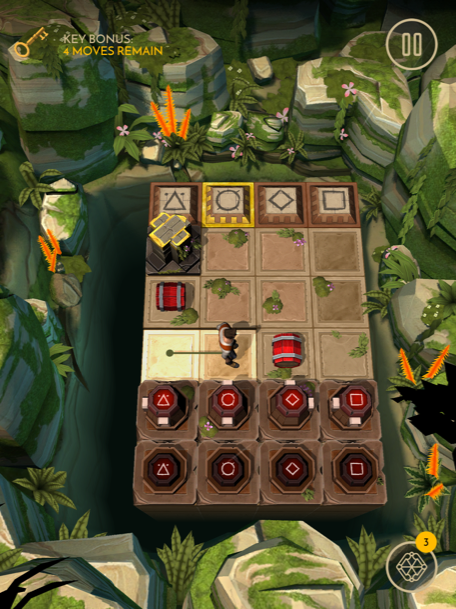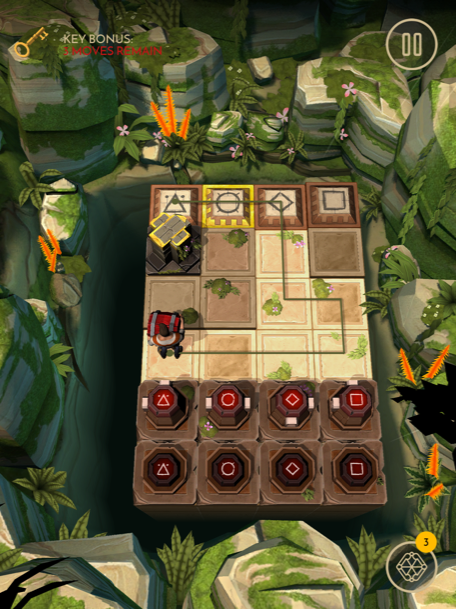Drop the bomb on the switch and grab the treasure.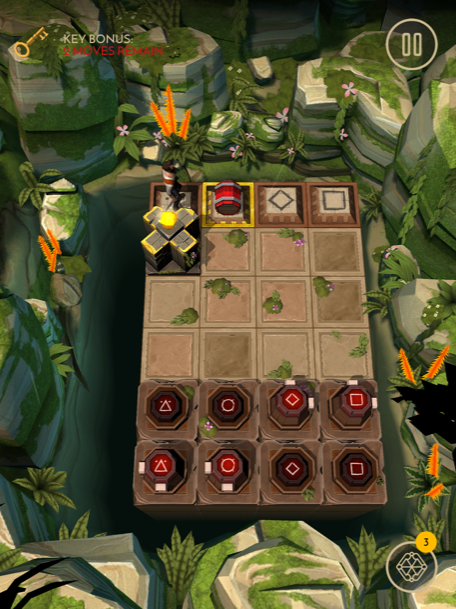Level 34

Move here and push the switch.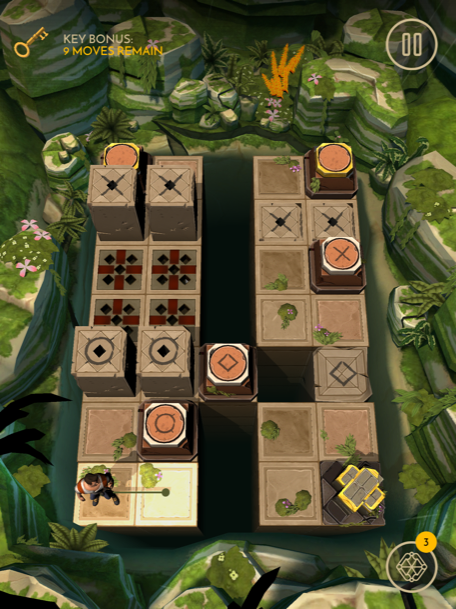Now move here and push the switch.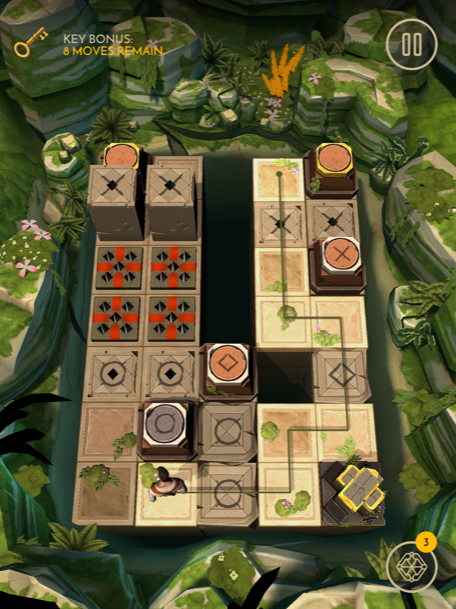Move here and push the switch.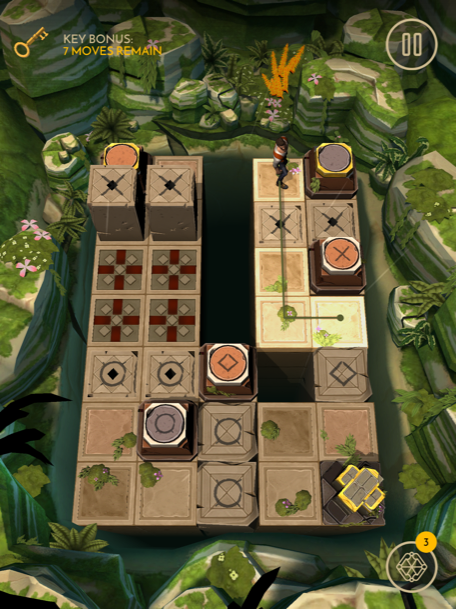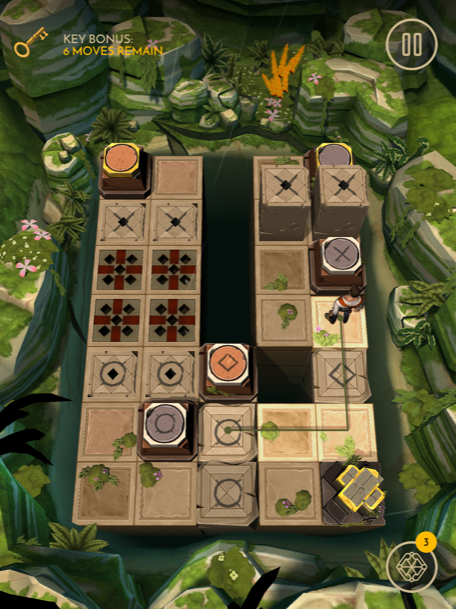Now follow this path and push the switch.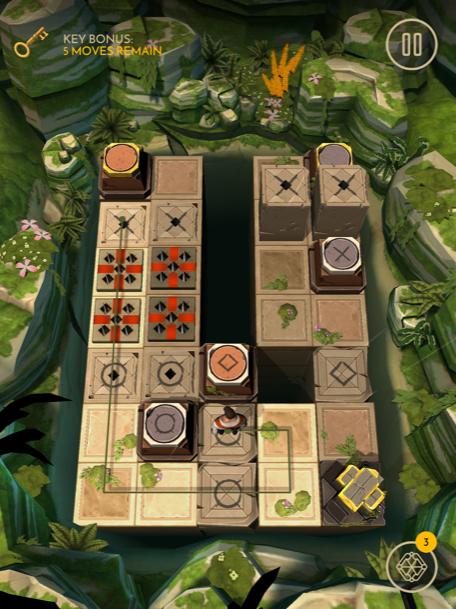Follow this path to the treasure.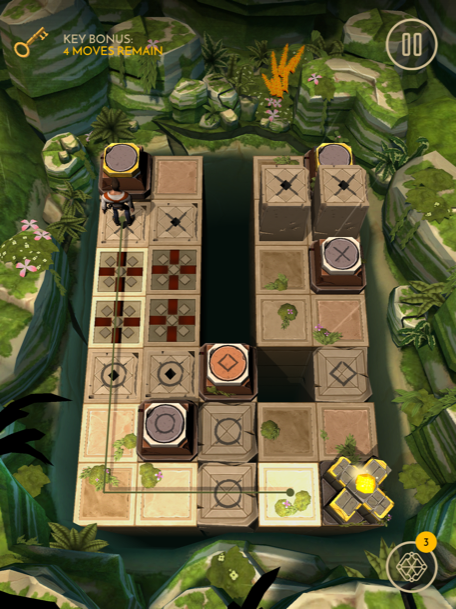Level 35

First move here.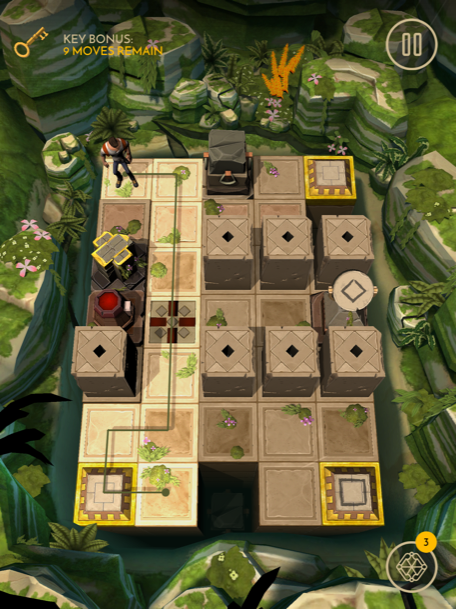Tap the block and push it here.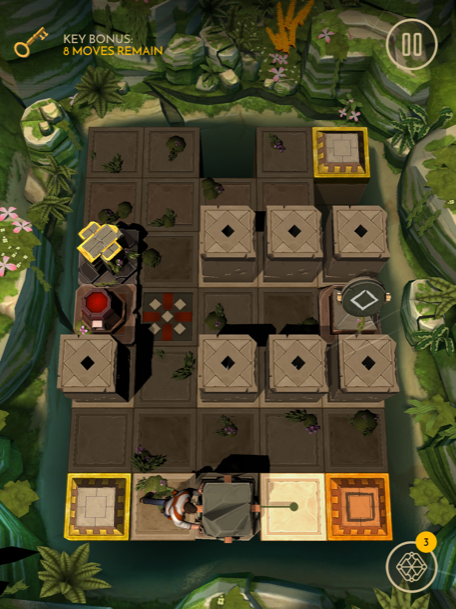Now move here.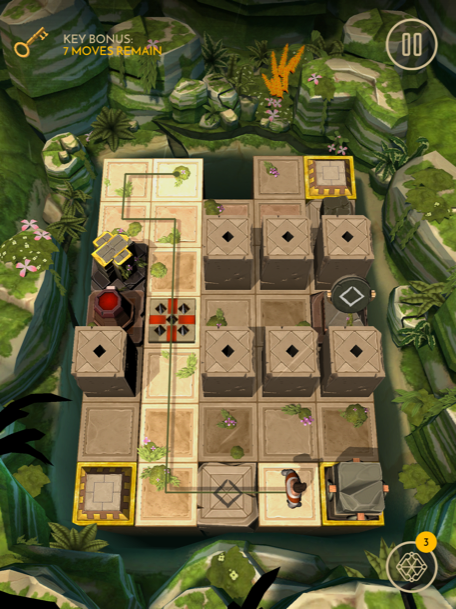Tap the block and push it here.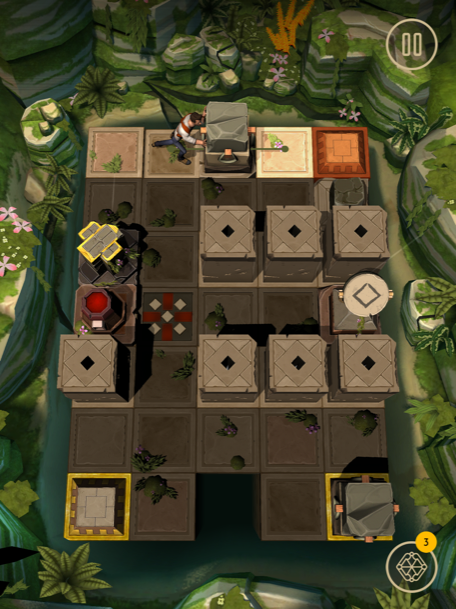Move here and pick up the small block.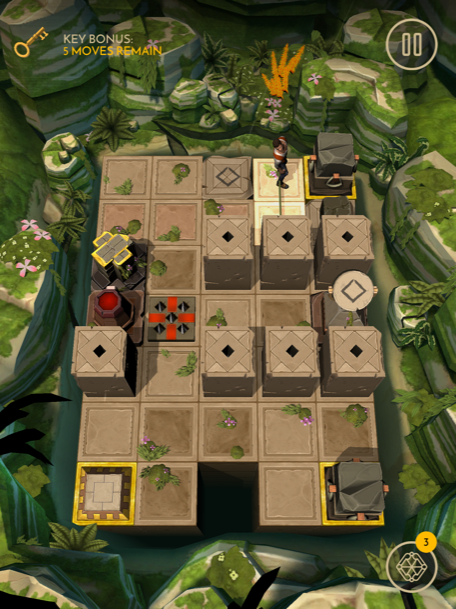Follow this path and drop the block on the switch.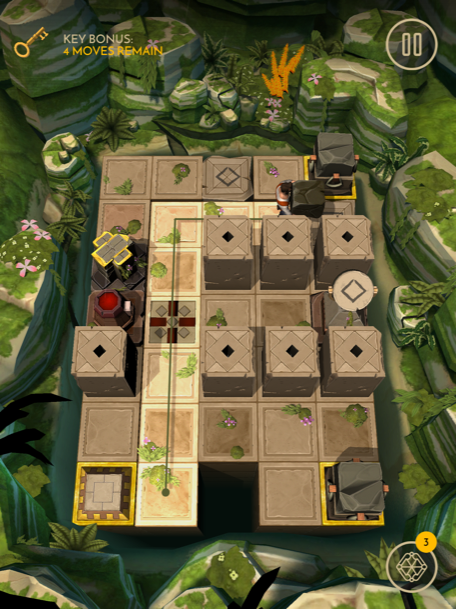Now follow this path and grab the treasure.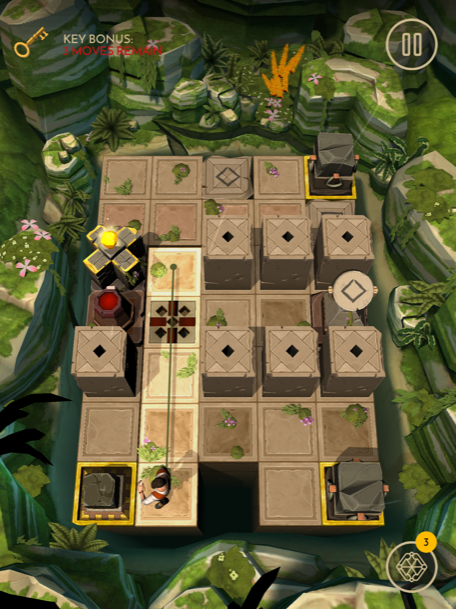Level 36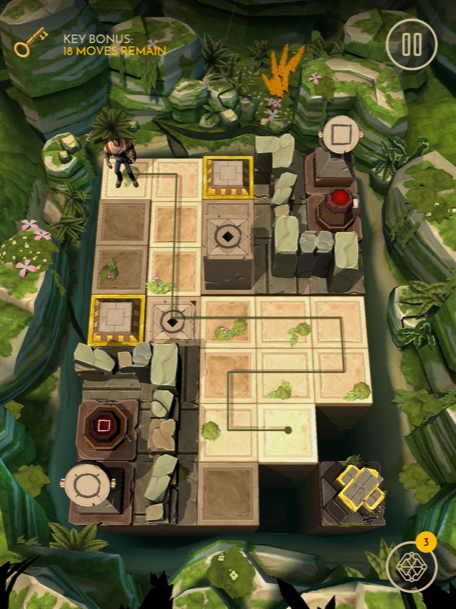Pick up the block, then follow this path.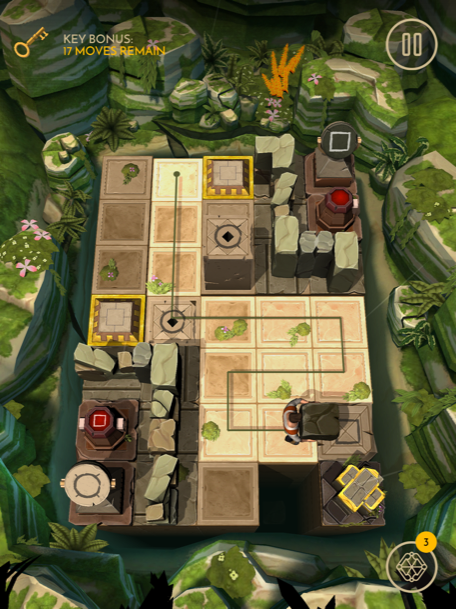Drop the block on the switch, then move here.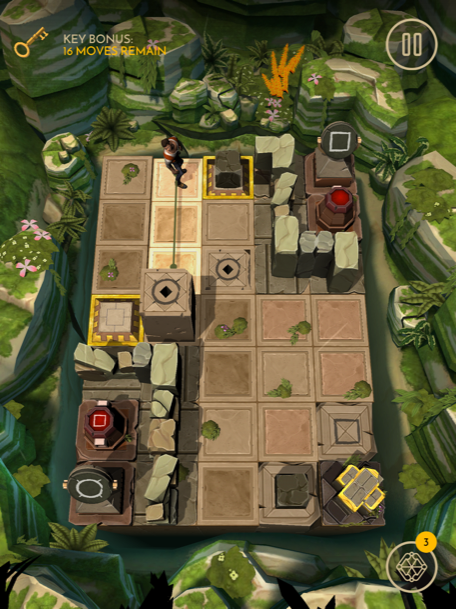Follow this path, then pick up the block.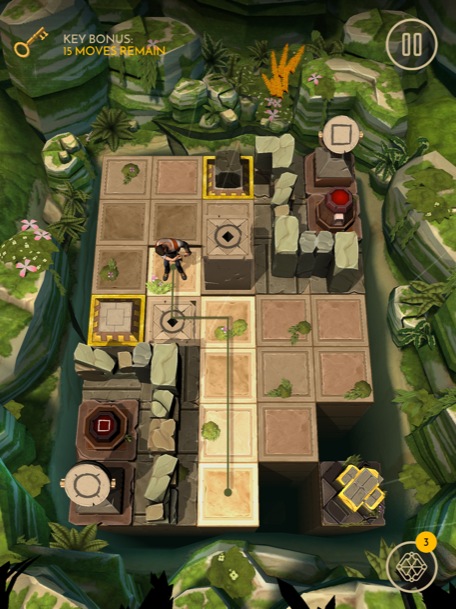Move here.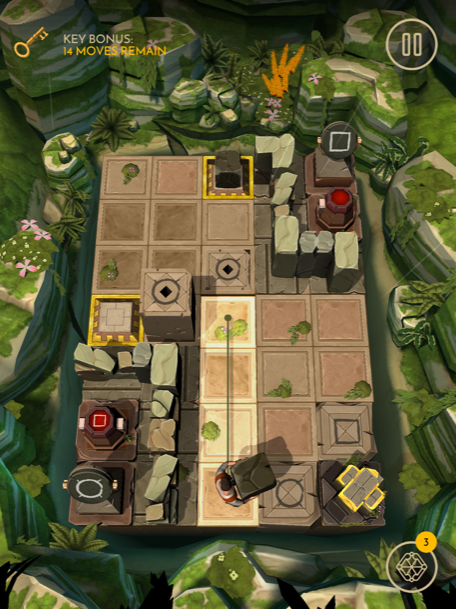Now move here and drop the block on the switch.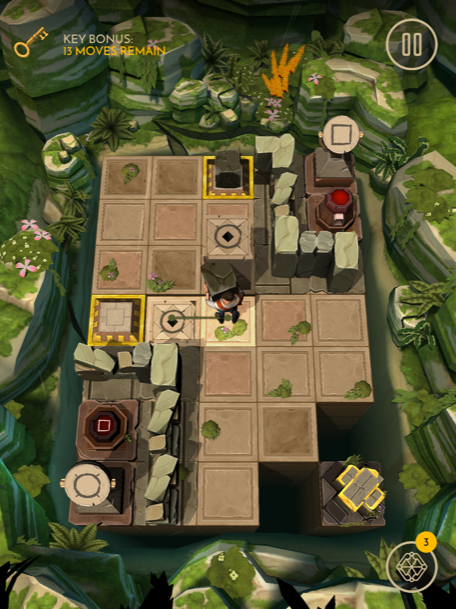Now move here.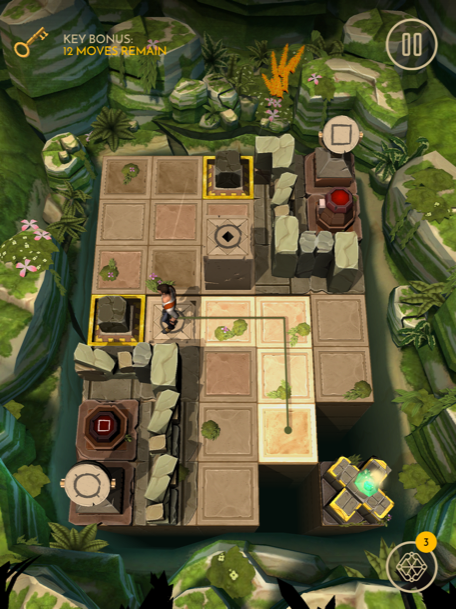Then move here and grab the treasure.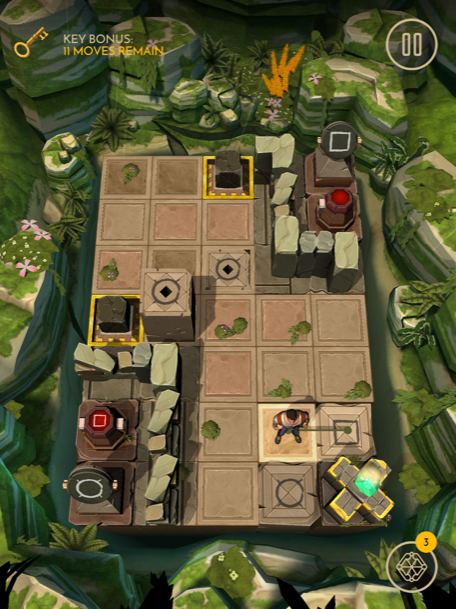Level 37

First move here.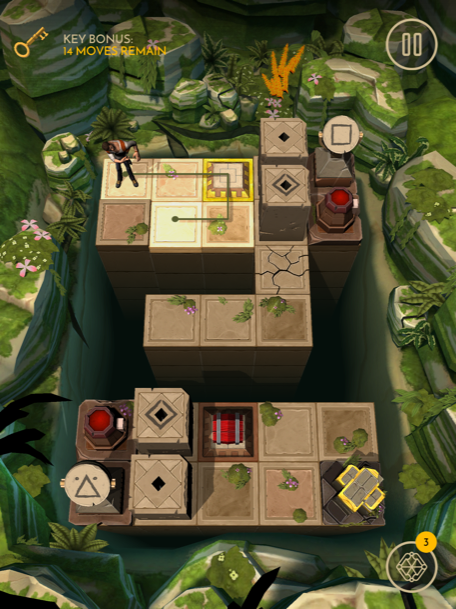Then move here.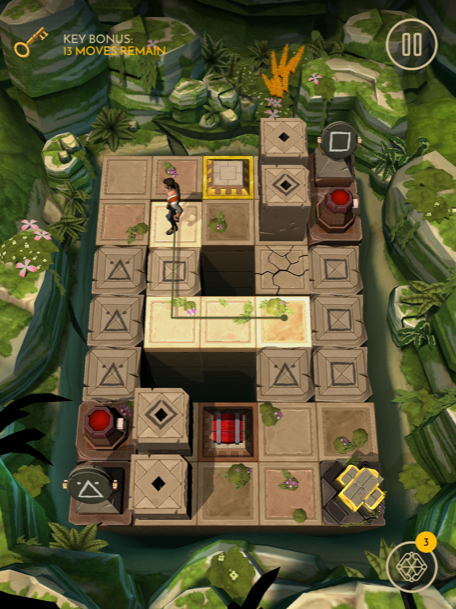Now move here.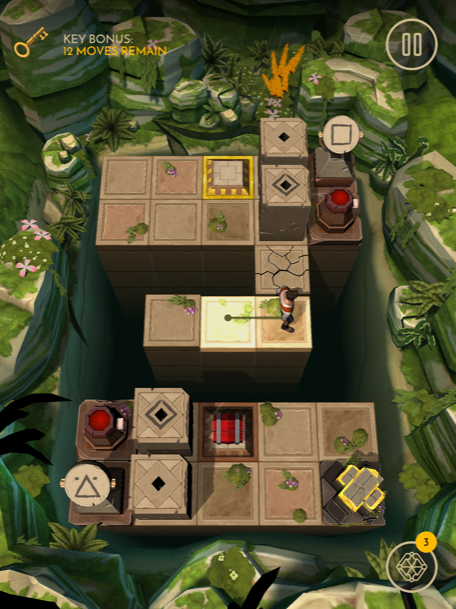And then back here.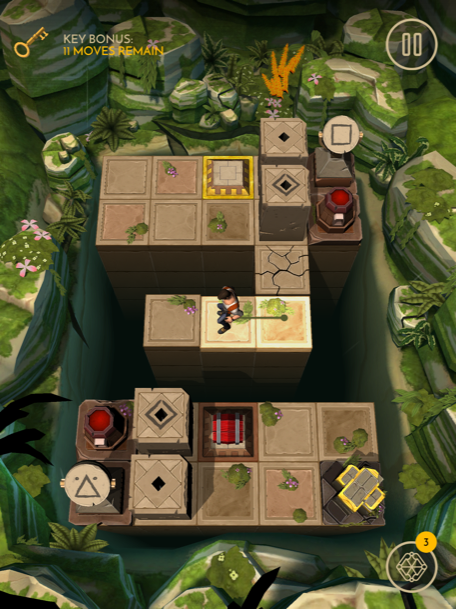Head here and grab the bomb.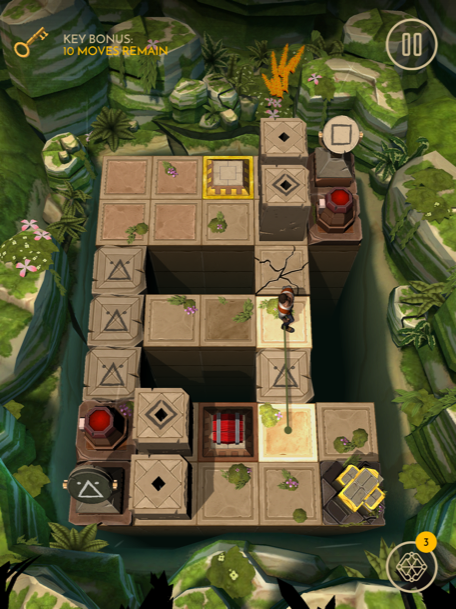Move here and drop the bomb on the switch.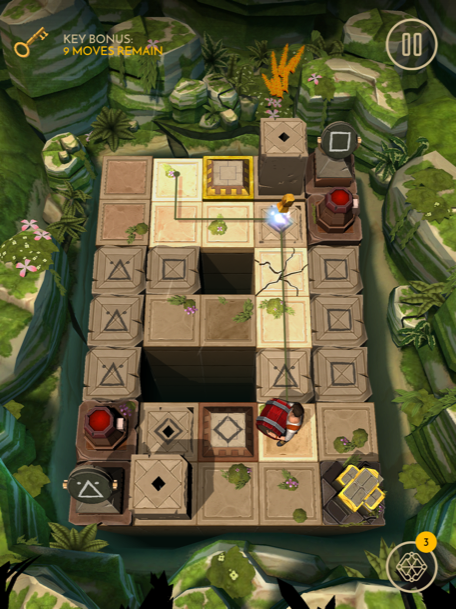Now move here.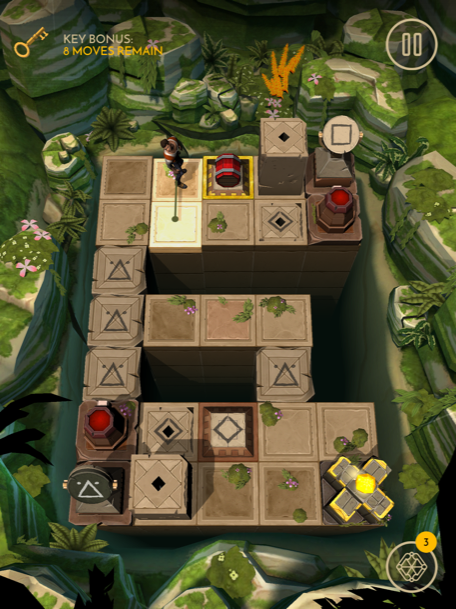Move here.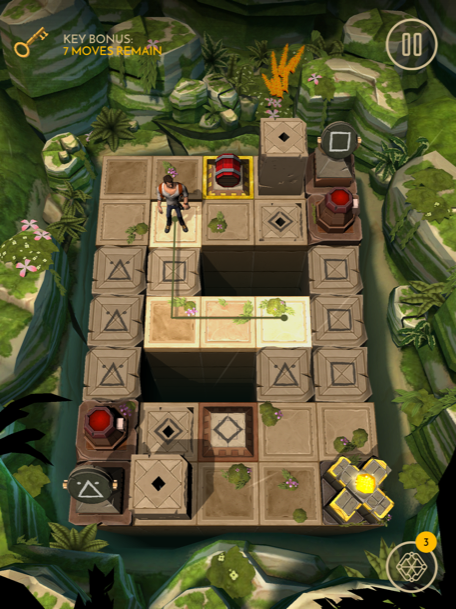Then move here.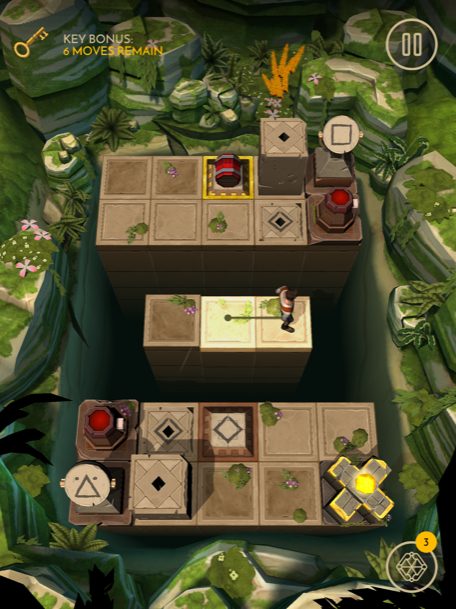Then back here again.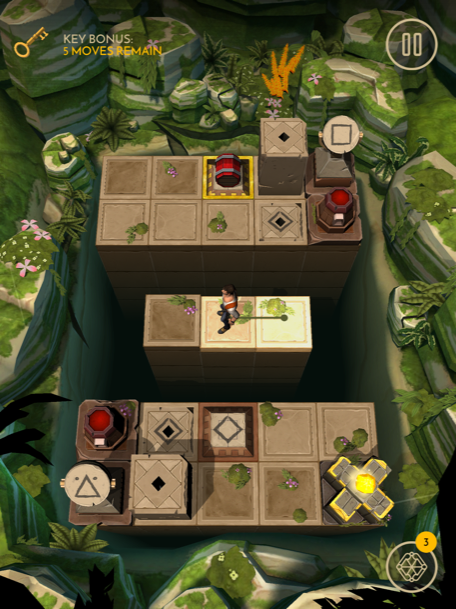Now follow this path and grab the treasure.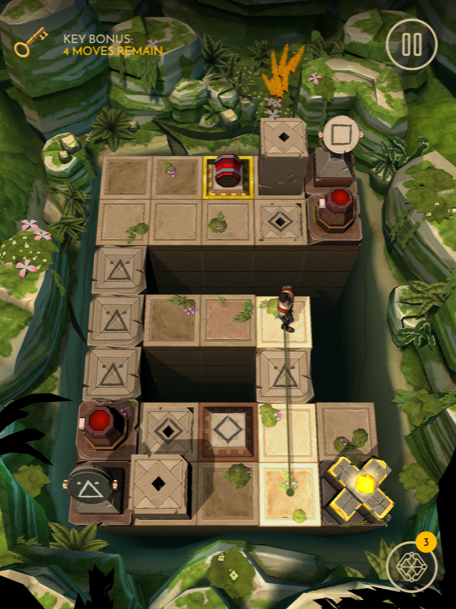Level 38

Pick up the bomb, put it on the switch, then move here.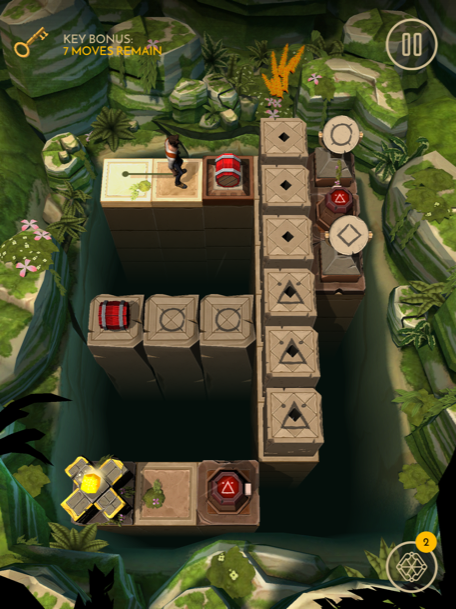Then move here.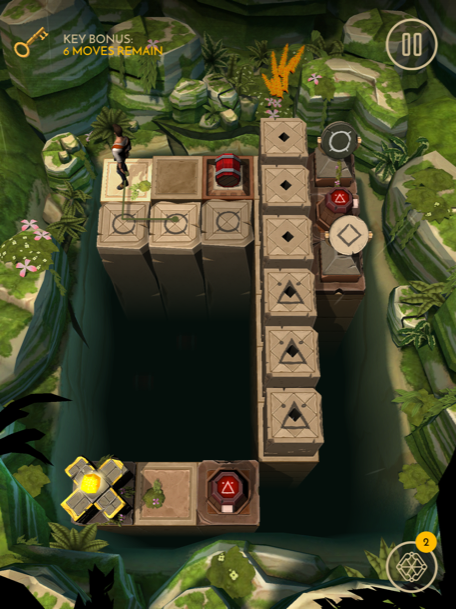Move here and pick up the bomb.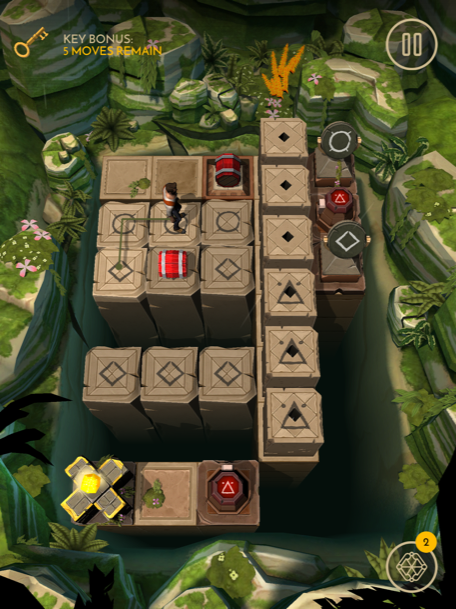Move here and put the bomb on the switch.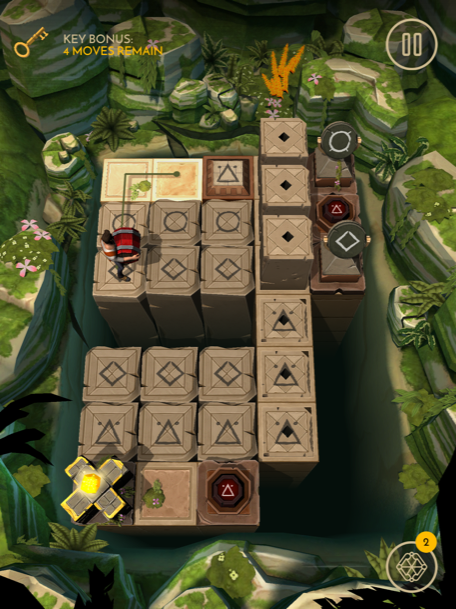Then move here.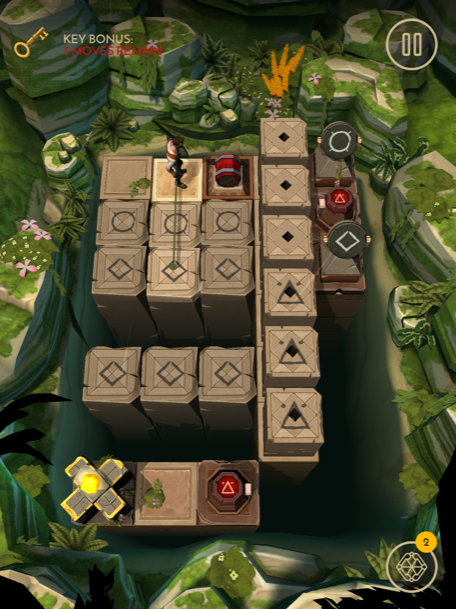Shoot the bomb on the switch, then move here and grab the treasure.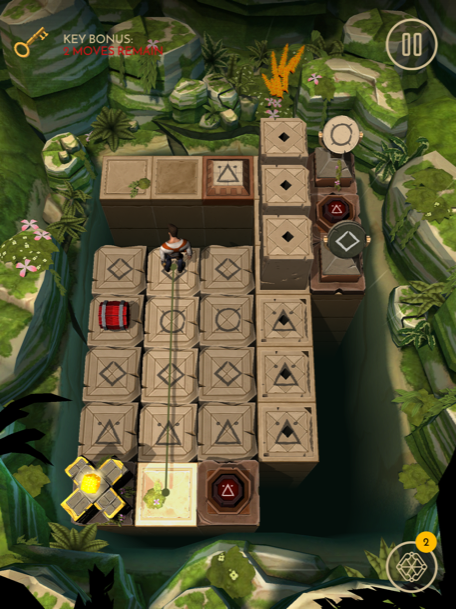Level 39

Move here and push the switch.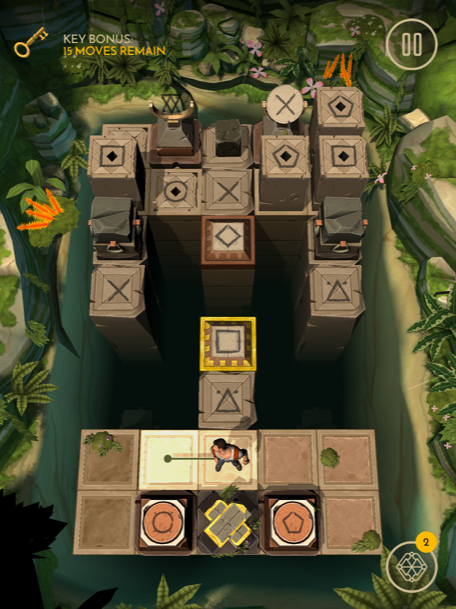Then move here and push the switch.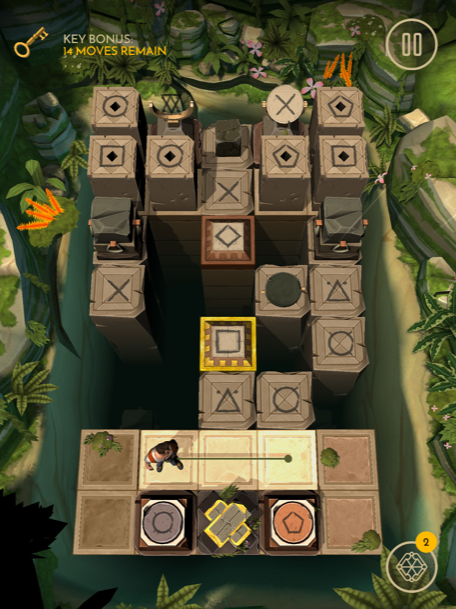Shoot the plate, then move here.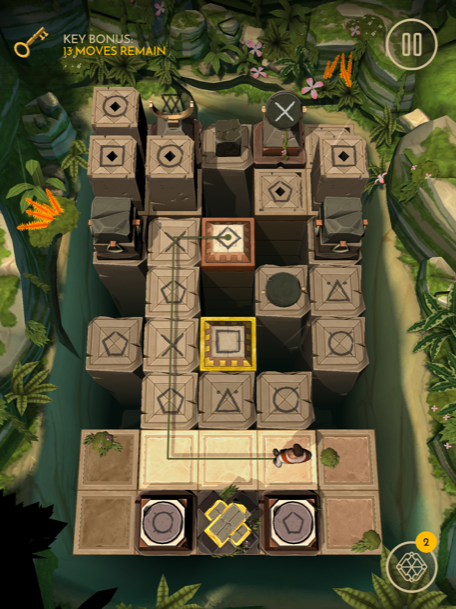Shoot the plate, then move here.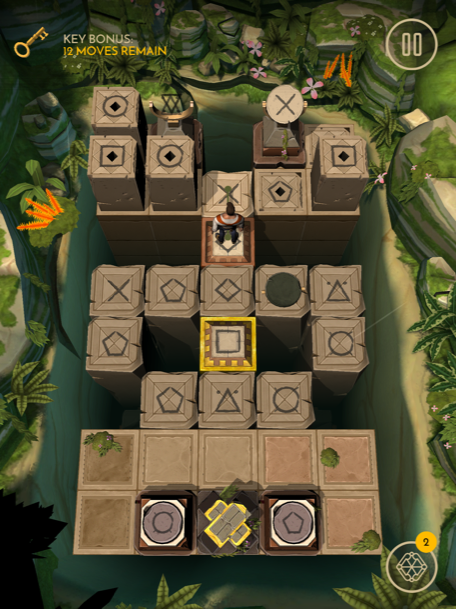Pick up the block, then move here.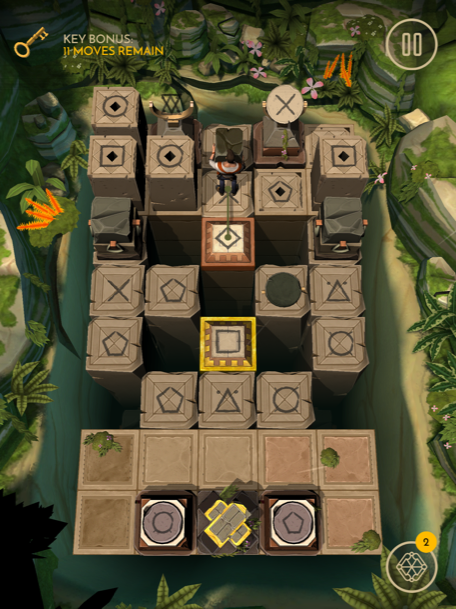Put the block down in front of you, shoot the plate, pick up the block, then move here.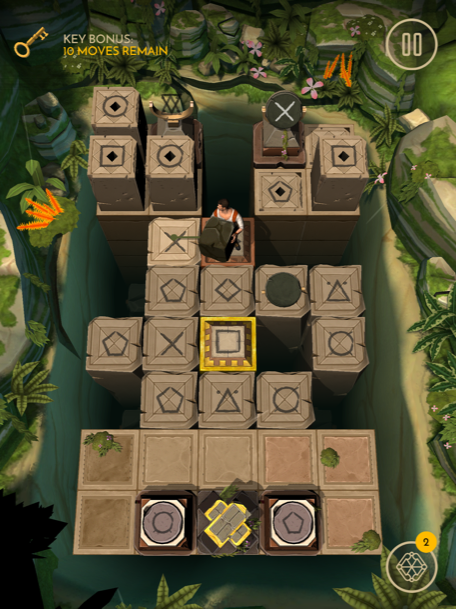Put the block on the switch, then move here.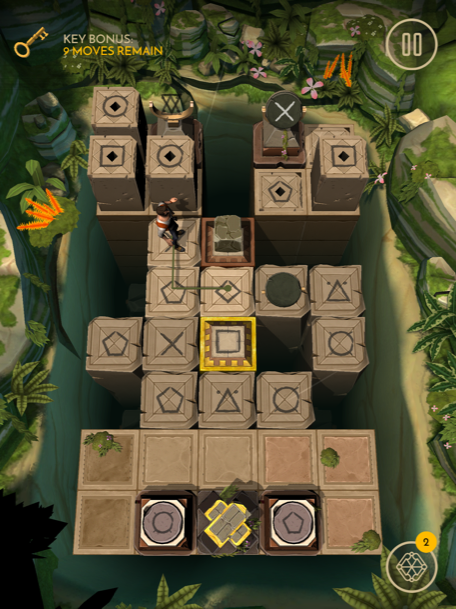Pick up the broken plate, then move here.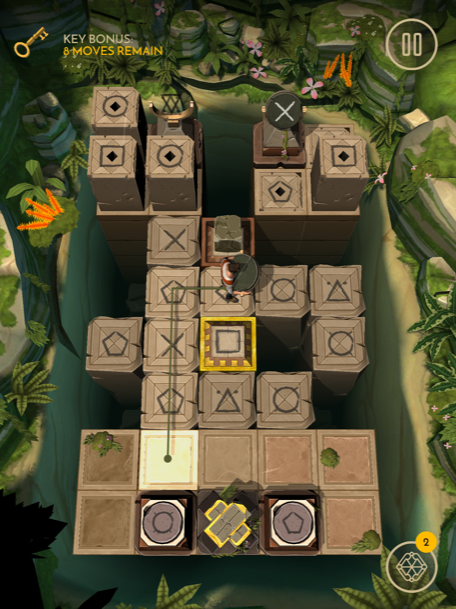Put the plate down, push the switch, pick up the plate again, then move here.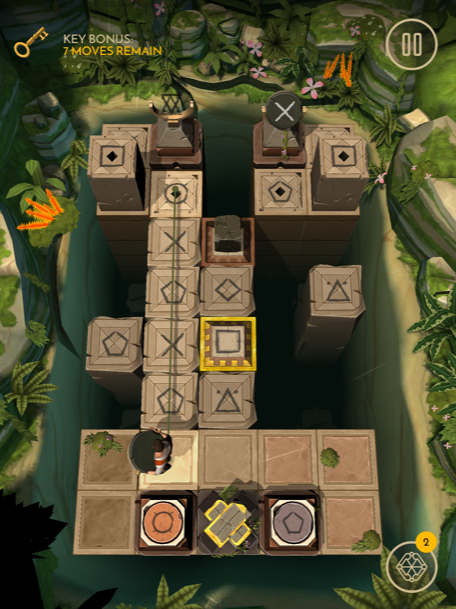Tap the cradle to fix the plate, shoot it, then move here.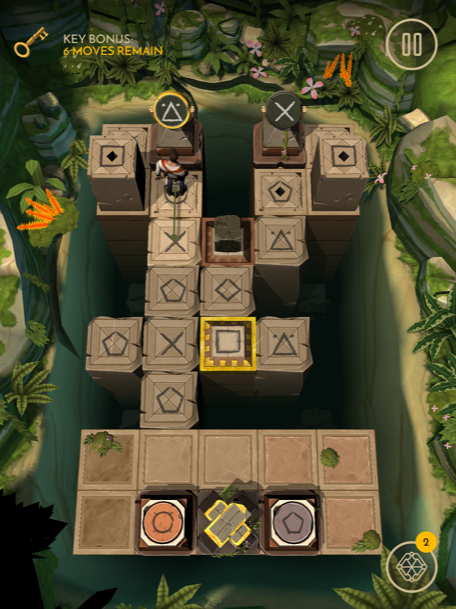Pick up the block, then move here.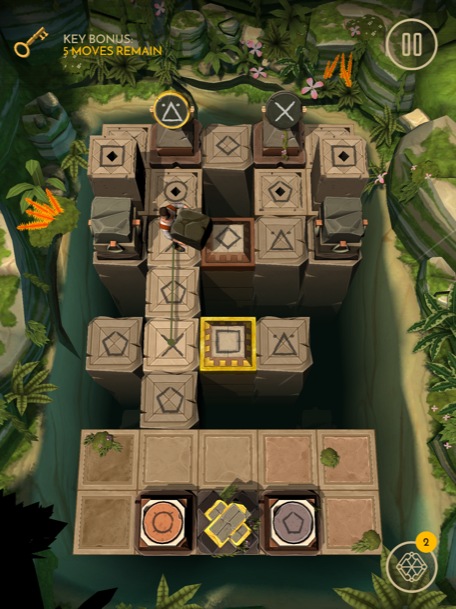Put the block on the switch, then follow this path to grab the treasure.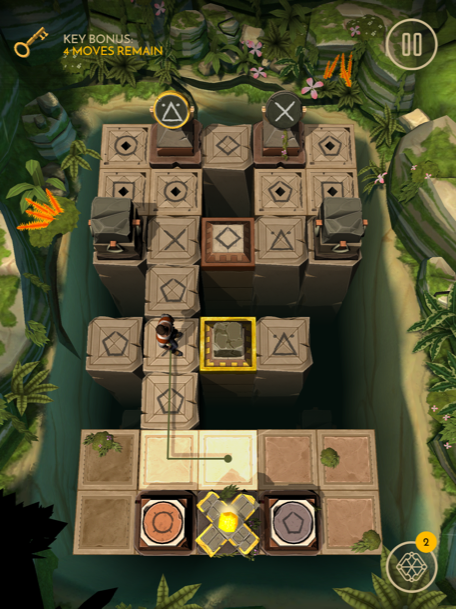Level 40

First move here and pick up the bomb.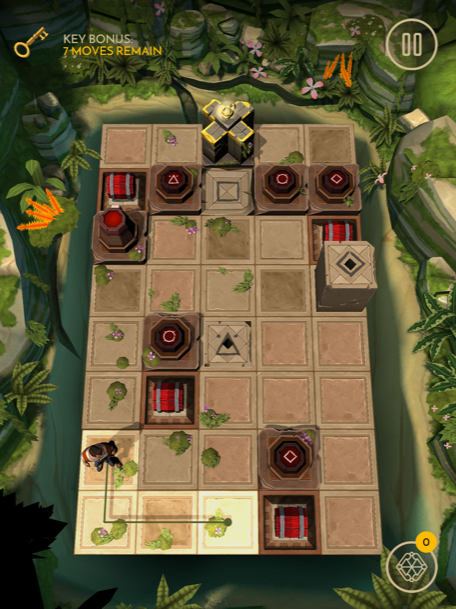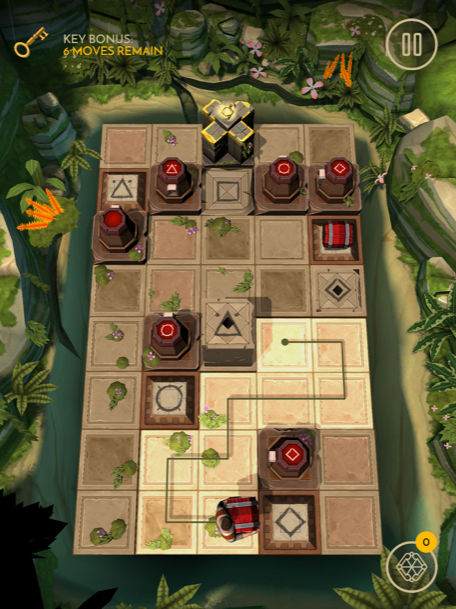Now move here.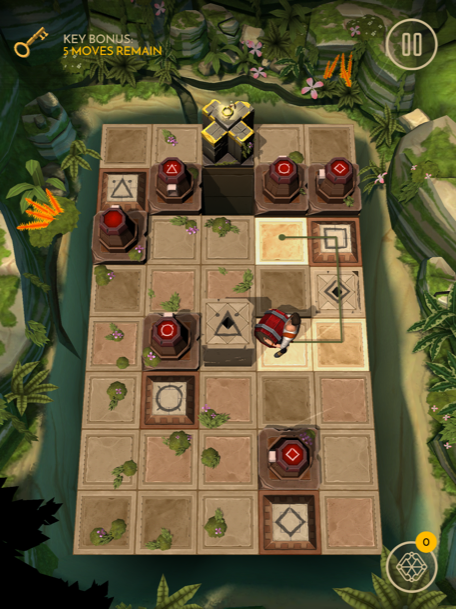Put the bomb on the switch, then move here to get the treasure.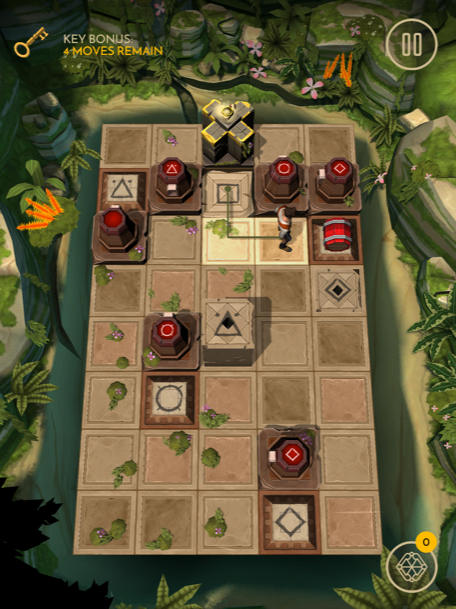Levels 1-10
Levels 11-20
Levels 21-30
Harry Slater
Harry used to be really good at Snake on the Nokia 5110. Apparently though, digital snake wrangling isn't a proper job, so now he writes words about games instead.